# How to Create and Customize Venn Diagrams in Python?

Venn Diagrams are useful for illustrating relations between two or more groups. We can easily see commonalities and differences between different groups. In this article, we are going to discuss how to create and customize Venn diagrams in Python:

Simple Venn Diagram: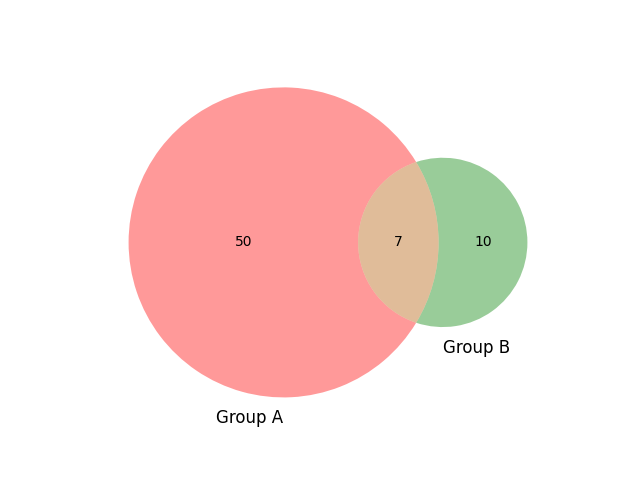Installation:

Install matplotlib-venn Library in your computer (Here we used the tool Pycharm) go to the terminal and use the following command.

`pip install matplotlib-venn`

After installing the library create a new python file and import the libraries as explained in the below program:

## Python3

 `# import modules ` `from` `matplotlib_venn ``import` `venn2  ` `from` `matplotlib ``import` `pyplot as plt ` ` `  `# depict venn diagram ` `venn2(subsets ``=` `(``50``, ``10``, ``7``), set_labels ``=` `(``'Group A'``, ``'Group B'``)) ` `plt.show()`

Output:The statement venn2(subsets = (30, 10, 5), set_labels = (‘Group A’, ‘Group B’)) refers to the subset’s parameter is a 3 element list where the numbers 50, 10, 7 correspond to Ab, aB, AB.

Ab = Contained in group A, but not B

aB = Contained in group B, but not A

AB = Contained in both group A and B

The set_labels parameter allows you to label your two groups in the Venn diagram. The show() function in pyplot module of matplotlib library is used to display all figures.

Below are various examples that depict how to create and customize Venn diagrams:

Example 1:

Venn Diagrams automatically size the circle depending upon the magnitude of items allotted. However, we can disable this by using an unweighted Venn Diagram, so the circles appear in the same size irrespective of the items allotted.

The default colors of Venn Diagrams are red and green now we will customize the colors orange and blue using set_colors parameter. The alpha parameter is used to control the transparency.

## Python3

 `# import modules ` `from` `matplotlib_venn ``import` `venn2_unweighted  ` `from` `matplotlib ``import` `pyplot as plt ` ` `  `# depict venn diagram ` `venn2_unweighted(subsets ``=` `(``50``, ``10``, ``7``), ` `                 ``set_labels ``=` `(``'Group A'``,  ` `                               ``'Group B'``), ` `                 ``set_colors``=``(``"orange"``, ` `                             ``"blue"``),alpha``=``0.7``) ` `plt.show()`

Output: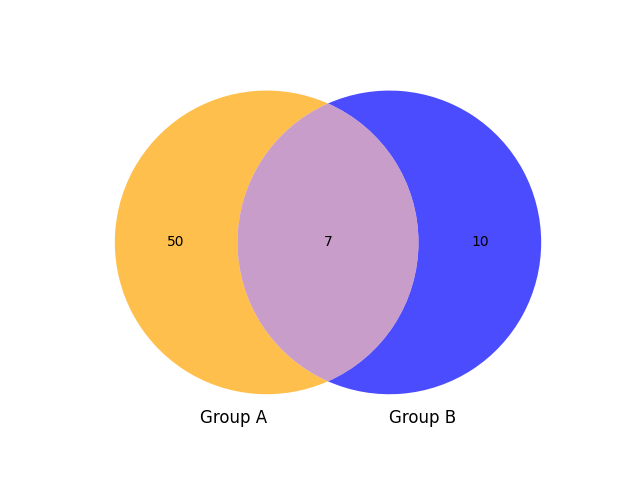Example 2:

We can customize the outline of the circle note it works on weighted Venn Diagrams which are shown in the below program.

## Python3

 `# import modules ` `from` `matplotlib_venn ``import` `venn2,venn2_circles ` `from` `matplotlib ``import` `pyplot as plt ` ` `  `# depict venn diagram ` `venn2(subsets ``=` `(``50``, ``10``, ``7``), ` `      ``set_labels ``=` `(``'Group A'``,  ` `                    ``'Group B'``), ` `      ``set_colors``=``(``"orange"``, ` `                  ``"blue"``),alpha``=``0.7``) ` ` `  `# add outline ` `venn2_circles(subsets``=``(``50``,``10``,``7``))  ` `plt.show()`

Output: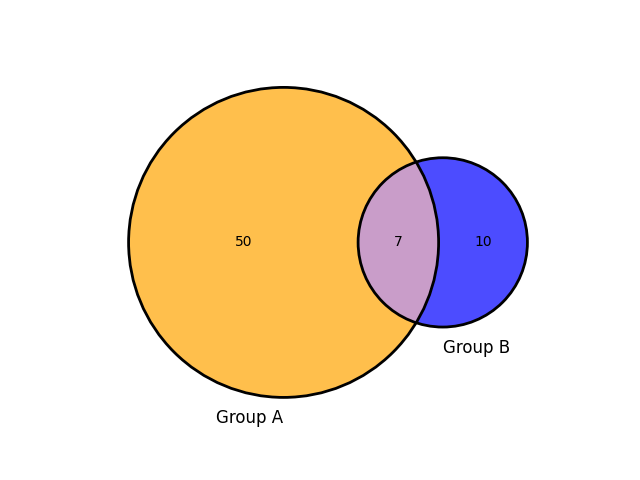Example 3:

We can also customize the outline of the circle with dashed line style and line width:

## Python3

 `# import modules ` `from` `matplotlib_venn ``import` `venn2, venn2_circles ` `from` `matplotlib ``import` `pyplot as plt ` ` `  `# depict venn diagram ` `venn2(subsets``=``(``50``, ``10``, ``7``),  ` `      ``set_labels``=``(``'Group A'``, ``'Group B'``), ` `      ``set_colors``=``(``"orange"``, ``"blue"``), alpha``=``0.7``) ` ` `  `# outline of the circle with defined  ` `# line style and line width ` `venn2_circles(subsets``=``(``50``, ``10``, ``7``),  ` `              ``linestyle``=``"dashed"``, linewidth``=``2``) ` `plt.show() `

Output: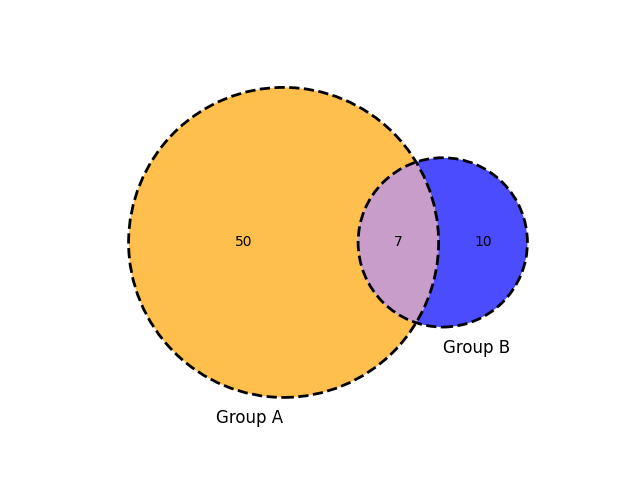Example 4:

A title can be assigned to Venn diagrams using the title() method.

## Python3

 `# import modules ` `from` `matplotlib_venn ``import` `venn2, venn2_circles ` `from` `matplotlib ``import` `pyplot as plt ` ` `  `# depict venn diagram ` `venn2(subsets``=``(``50``, ``10``, ``7``),  ` `      ``set_labels``=``(``'Group A'``, ``'Group B'``), ` `      ``set_colors``=``(``"orange"``, ``"blue"``), alpha``=``0.7``) ` ` `  `# add outline ` `venn2_circles(subsets``=``(``50``, ``10``, ``7``),  ` `              ``linestyle``=``"dashed"``,  ` `              ``linewidth``=``2``) ` ` `  `# assign title of the venn diagram ` `plt.title(``"Venn Diagram in geeks for geeks"``)   ` `plt.show() `

Output: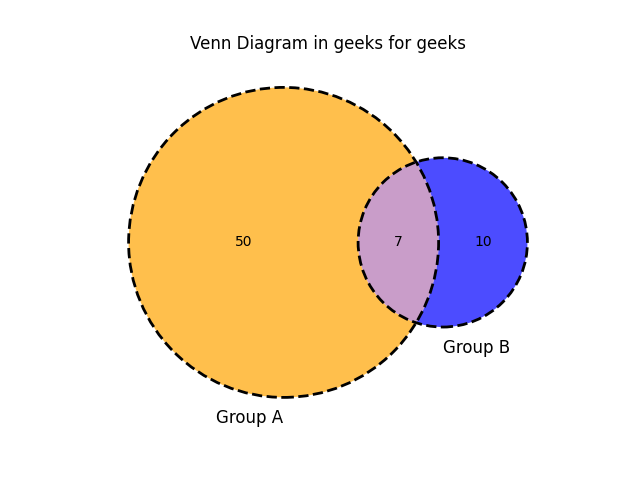Example 6:

Let us draw three Venn Diagrams use venn3, venn3_circles modules.

## Python3

 `# import module ` `from` `matplotlib_venn ``import` `venn3, venn3_circles ` `from` `matplotlib ``import` `pyplot as plt ` ` `  `# depict venn diagram ` `venn3(subsets``=``(``20``, ``10``, ``12``, ``10``, ``9``, ``4``, ``3``),  ` `      ``set_labels``=``(``'Group A'``, ``'Group B'``, ``'Group C'``),  ` `      ``set_colors``=``(``"orange"``, ``"blue"``, ``"red"``), alpha``=``0.7``) ` ` `  `# outline of circle line style and width ` `venn3_circles(subsets``=``(``20``, ``10``, ``12``, ``10``, ``9``, ``4``, ``3``), ` `              ``linestyle``=``"dashed"``, linewidth``=``2``) ` ` `  `# title of the venn diagram ` `plt.title(``"Venn Diagram in geeks for geeks"``) ` `plt.show() `

Output: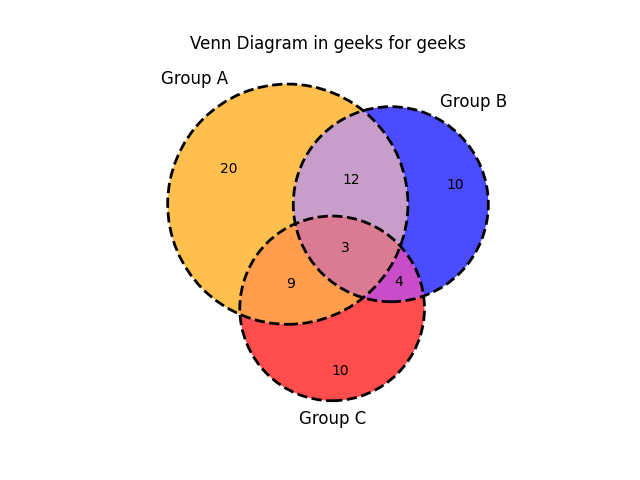Example 7:

Let us customize the colors of each area of the diagram with the get_patch_by_id() method.

## Python3

 `#import module ` `from` `matplotlib_venn ``import` `venn3, venn3_circles ` `from` `matplotlib ``import` `pyplot as plt ` ` `  `# depict venn diagram ` `v ``=` `venn3(subsets``=``(``1``, ``1``, ``1``, ``1``, ``1``, ``1``, ``1``),  ` `          ``set_labels``=``(``'A'``, ``'B'``, ``'C'``)) ` ` `  `# set color to defined path id ` `v.get_patch_by_id(``"100"``).set_color(``"white"``) ` `# set text to defined label id ` `v.get_label_by_id(``"100"``).set_text(``"unknown"``) ` `# set text to defined label id "A" ` `v.get_label_by_id(``'A'``).set_text(``'A new'``) ` ` `  `# add outline ` `venn3_circles(subsets``=``(``1``, ``1``, ``1``, ``1``, ``1``, ``1``, ``1``),  ` `              ``linestyle``=``"dashed"``, linewidth``=``2``) ` ` `  `# assign title ` `plt.title(``"Venn Diagram in geeks for geeks"``) ` `plt.show() `

Output: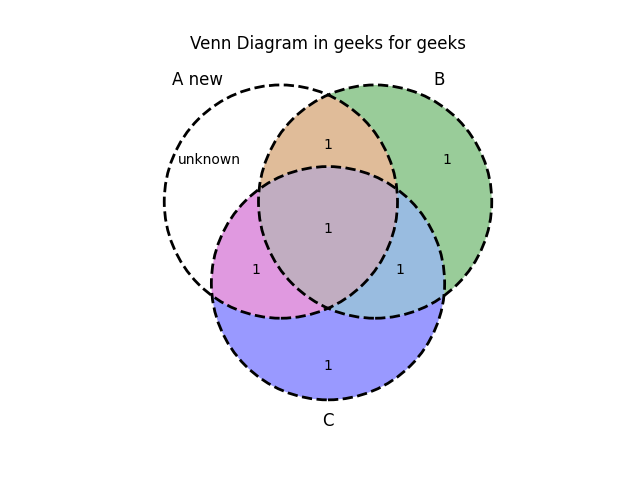Whether you're preparing for your first job interview or aiming to upskill in this ever-evolving tech landscape, GeeksforGeeks Courses are your key to success. We provide top-quality content at affordable prices, all geared towards accelerating your growth in a time-bound manner. Join the millions we've already empowered, and we're here to do the same for you. Don't miss out - check it out now!

Previous
Next